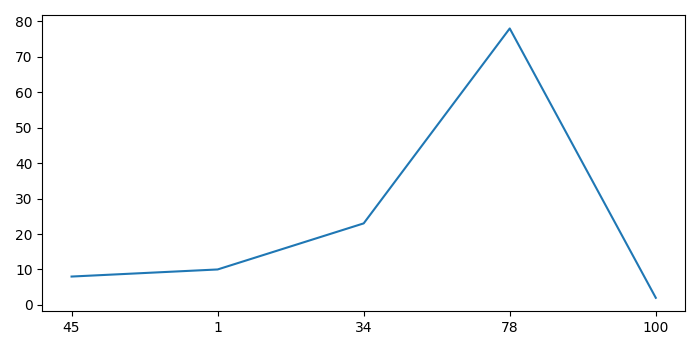# How to set X-axis values in Matplotlib Python?

To set X-axis values in matplotlib in Python, we can take the following steps −

• Create two lists for x and y data points.

• Get the xticks range value.

• Plot a line using plot() method with xtick range value and y data points.

• Replace xticks with X-axis value using xticks() method.

• To display the figure, use show() method.

## Example

from matplotlib import pyplot as plt
plt.rcParams["figure.figsize"] = [7.00, 3.50]
plt.rcParams["figure.autolayout"] = True
x = [45, 1, 34, 78, 100]
y = [8, 10, 23, 78, 2]
default_x_ticks = range(len(x))
plt.plot(default_x_ticks, y)
plt.xticks(default_x_ticks, x)
plt.show()

## Output